Logic gate circuits are designed to input and output only two types of signals: “high” (1) and “low” (0), as represented by a variable voltage: full power supply voltage for a “high” state and zero voltage for a “low” state. In a perfect world, all logic circuit signals would exist at these extreme voltage limits, and never deviate from them (i.e., less than full voltage for a “high,” or more than zero voltage for a “low”). However, in reality, logic signal voltage levels rarely attain these perfect limits due to stray voltage drops in the transistor circuitry, and so we must understand the signal level limitations of gate circuits as they try to interpret signal voltages lying somewhere between full supply voltage and zero.
TTL gates operate on a nominal power supply voltage of 5 volts, +/- 0.25 volts. Ideally, a TTL “high” signal would be 5.00 volts exactly, and a TTL “low” signal 0.00 volts exactly. However, real TTL gate circuits cannot output such perfect voltage levels, and are designed to accept “high” and “low” signals deviating substantially from these ideal values. “Acceptable” input signal voltages range from 0 volts to 0.8 volts for a “low” logic state, and 2 volts to 5 volts for a “high” logic state. “Acceptable” output signal voltages (voltage levels guaranteed by the gate manufacturer over a specified range of load conditions) range from 0 volts to 0.5 volts for a “low” logic state, and 2.7 volts to 5 volts for a “high” logic state: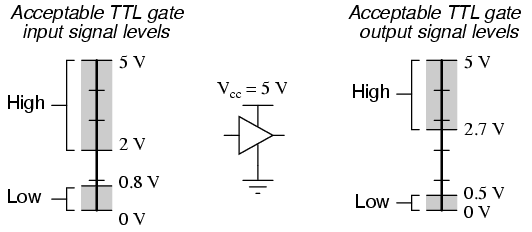If a voltage signal ranging between 0.8 volts and 2 volts were to be sent into the input of a TTL gate, there would be no certain response from the gate. Such a signal would be considered uncertain, and no logic gate manufacturer would guarantee how their gate circuit would interpret such a signal.
As you can see, the tolerable ranges for output signal levels are narrower than for input signal levels, to ensure that any TTL gate outputting a digital signal into the input of another TTL gate will transmit voltages acceptable to the receiving gate. The difference between the tolerable output and input ranges is called the noise margin of the gate. For TTL gates, the low-level noise margin is the difference between 0.8 volts and 0.5 volts (0.3 volts), while the high-level noise margin is the difference between 2.7 volts and 2 volts (0.7 volts). Simply put, the noise margin is the peak amount of spurious or “noise” voltage that may be superimposed on a weak gate output voltage signal before the receiving gate might interpret it wrongly: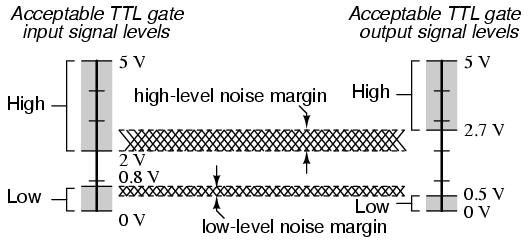CMOS gate circuits have input and output signal specifications that are quite different from TTL. For a CMOS gate operating at a power supply voltage of 5 volts, the acceptable input signal voltages range from 0 volts to 1.5 volts for a “low” logic state, and 3.5 volts to 5 volts for a “high” logic state. “Acceptable” output signal voltages (voltage levels guaranteed by the gate manufacturer over a specified range of load conditions) range from 0 volts to 0.05 volts for a “low” logic state, and 4.95 volts to 5 volts for a “high” logic state: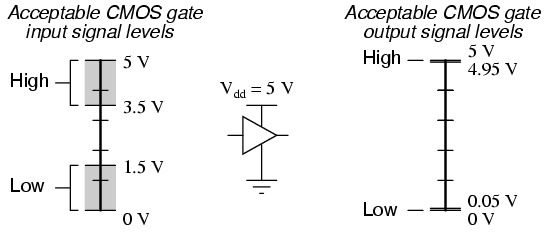It should be obvious from these figures that CMOS gate circuits have far greater noise margins than TTL: 1.45 volts for CMOS low-level and high-level margins, versus a maximum of 0.7 volts for TTL. In other words, CMOS circuits can tolerate over twice the amount of superimposed “noise” voltage on their input lines before signal interpretation errors will result.
CMOS noise margins widen even further with higher operating voltages. Unlike TTL, which is restricted to a power supply voltage of 5 volts, CMOS may be powered by voltages as high as 15 volts (some CMOS circuits as high as 18 volts). Shown here are the acceptable “high” and “low” states, for both input and output, of CMOS integrated circuits operating at 10 volts and 15 volts, respectively: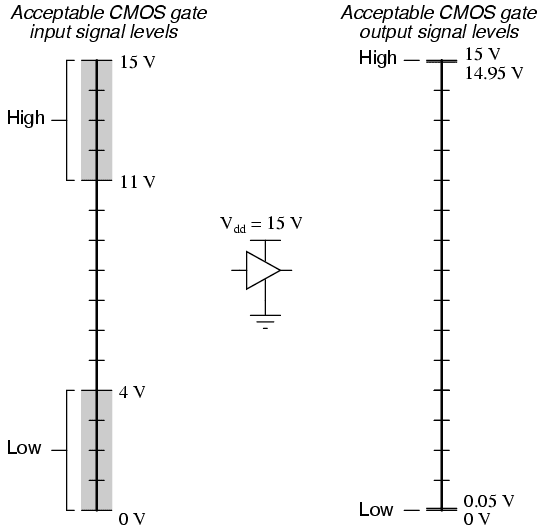The margins for acceptable “high” and “low” signals may be greater than what is shown in the previous illustrations. What is shown represents “worst-case” input signal performance, based on manufacturer’s specifications. In practice, it may be found that a gate circuit will tolerate “high” signals of considerably less voltage and “low” signals of considerably greater voltage than those specified here.
Conversely, the extremely small output margins shown—guaranteeing output states for “high” and “low” signals to within 0.05 volts of the power supply “rails”—are optimistic. Such “solid” output voltage levels will be true only for conditions of minimum loading. If the gate is sourcing or sinking substantial current to a load, the output voltage will not be able to maintain these optimum levels, due to internal channel resistance of the gate’s final output MOSFETs.
Within the “uncertain” range for any gate input, there will be some point of demarcation dividing the gate’s actual “low” input signal range from its actual “high” input signal range. That is, somewhere between the lowest “high” signal voltage level and the highest “low” signal voltage level guaranteed by the gate manufacturer, there is a threshold voltage at which the gate will actually switch its interpretation of a signal from “low” or “high” or vice versa. For most gate circuits, this unspecified voltage is a single point: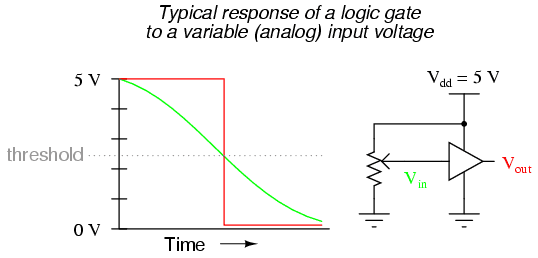In the presence of AC “noise” voltage superimposed on the DC input signal, a single threshold point at which the gate alters its interpretation of logic level will result in an erratic output: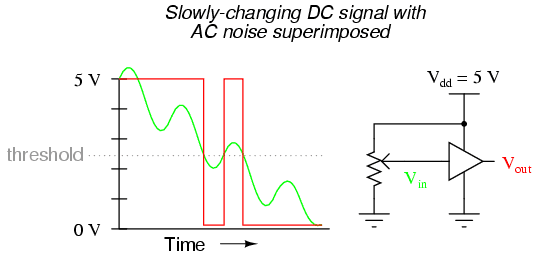If this scenario looks familiar to you, its because you remember a similar problem with (analog) voltage comparator op-amp circuits. With a single threshold point at which an input causes the output to switch between “high” and “low” states, the presence of significant noise will cause erratic changes in the output: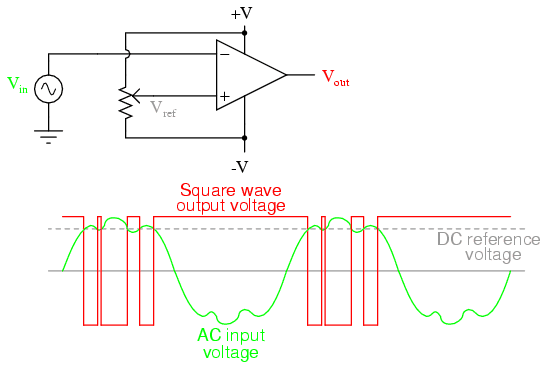The solution to this problem is a bit of positive feedback introduced into the amplifier circuit. With an op-amp, this is done by connecting the output back around to the noninverting (+) input through a resistor. In a gate circuit, this entails redesigning the internal gate circuitry, establishing the feedback inside the gate package rather than through external connections. A gate so designed is called a Schmitt trigger. Schmitt triggers interpret varying input voltages according totwo threshold voltages: a positive-going threshold (VT+), and a negative-going threshold (VT-):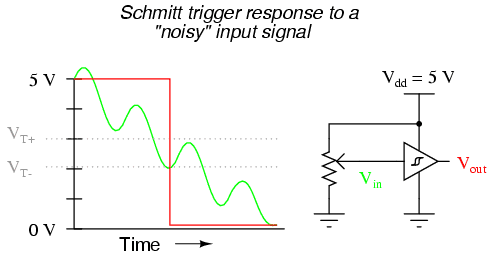Schmitt trigger gates are distinguished in schematic diagrams by the small “hysteresis” symbol drawn within them, reminiscent of the B-H curve for a ferromagnetic material. Hysteresis engendered by positive feedback within the gate circuitry adds an additional level of noise immunity to the gate’s performance. Schmitt trigger gates are frequently used in applications where noise is expected on the input signal line(s), and/or where an erratic output would be very detrimental to system performance.
The differing voltage level requirements of TTL and CMOS technology present problems when the two types of gates are used in the same system. Although operating CMOS gates on the same 5.00 volt power supply voltage required by the TTL gates is no problem, TTL output voltage levels will not be compatible with CMOS input voltage requirements.
Take for instance a TTL NAND gate outputting a signal into the input of a CMOS inverter gate. Both gates are powered by the same 5.00 volt supply (Vcc). If the TTL gate outputs a “low” signal (guaranteed to be between 0 volts and 0.5 volts), it will be properly interpreted by the CMOS gate’s input as a “low” (expecting a voltage between 0 volts and 1.5 volts):However, if the TTL gate outputs a “high” signal (guaranteed to be between 5 volts and 2.7 volts), it might not be properly interpreted by the CMOS gate’s input as a “high” (expecting a voltage between 5 volts and 3.5 volts):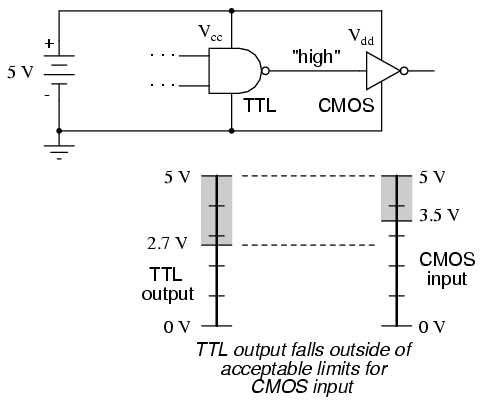Given this mismatch, it is entirely possible for the TTL gate to output a valid “high” signal (valid, that is, according to the standards for TTL) that lies within the “uncertain” range for the CMOS input, and may be (falsely) interpreted as a “low” by the receiving gate. An easy “fix” for this problem is to augment the TTL gate’s “high” signal voltage level by means of a pullup resistor: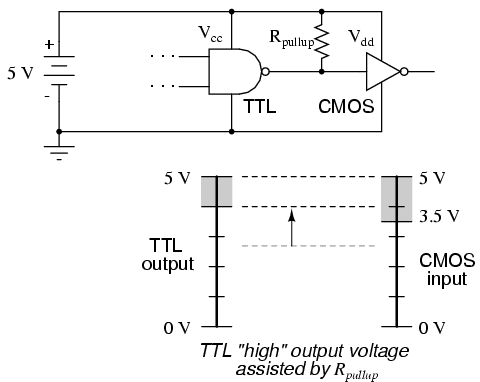Something more than this, though, is required to interface a TTL output with a CMOS input, if the receiving CMOS gate is powered by a greater power supply voltage: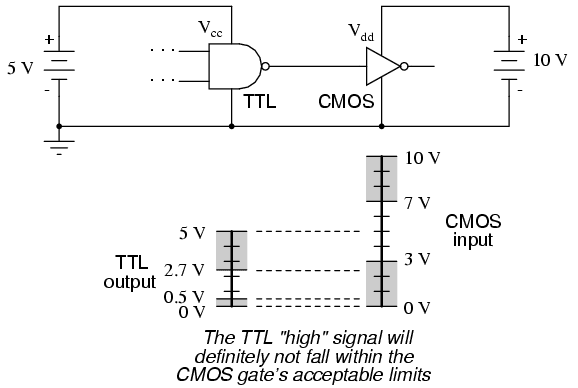There will be no problem with the CMOS gate interpreting the TTL gate’s “low” output, of course, but a “high” signal from the TTL gate is another matter entirely. The guaranteed output voltage range of 2.7 volts to 5 volts from the TTL gate output is nowhere near the CMOS gate’s acceptable range of 7 volts to 10 volts for a “high” signal. If we use an open-collector TTL gate instead of a totem-pole output gate, though, a pullup resistor to the 10 volt Vdd supply rail will raise the TTL gate’s “high” output voltage to the full power supply voltage supplying the CMOS gate. Since an open-collector gate can only sink current, not source current, the “high” state voltage level is entirely determined by the power supply to which the pullup resistor is attached, thus neatly solving the mismatch problem: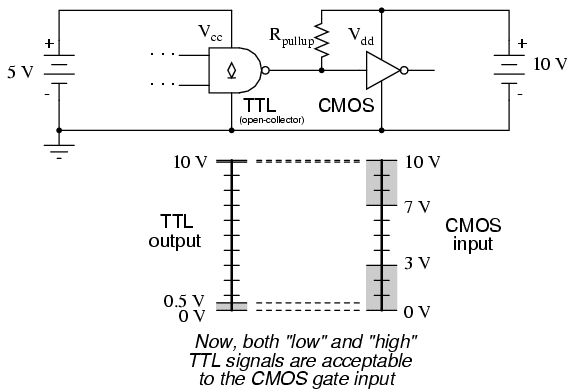Due to the excellent output voltage characteristics of CMOS gates, there is typically no problem connecting a CMOS output to a TTL input. The only significant issue is the current loading presented by the TTL inputs, since the CMOS output must sink current for each of the TTL inputs while in the “low” state.
When the CMOS gate in question is powered by a voltage source in excess of 5 volts (Vcc), though, a problem will result. The “high” output state of the CMOS gate, being greater than 5 volts, will exceed the TTL gate’s acceptable input limits for a “high” signal. A solution to this problem is to create an “open-collector” inverter circuit using a discrete NPN transistor, and use it to interface the two gates together: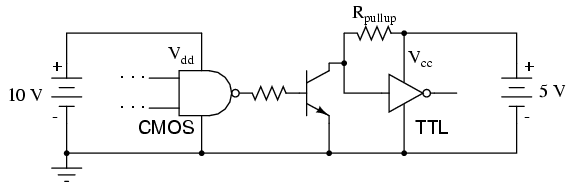The “Rpullup” resistor is optional, since TTL inputs automatically assume a “high” state when left floating, which is what will happen when the CMOS gate output is “low” and the transistor cuts off. Of course, one very important consequence of implementing this solution is the logical inversion created by the transistor: when the CMOS gate outputs a “low” signal, the TTL gate sees a “high” input; and when the CMOS gate outputs a “high” signal, the transistor saturates and the TTL gate sees a “low” input. So long as this inversion is accounted for in the logical scheme of the system, all will be well.
Logic signal levels in voltagesReviewed by Suresh Bojja on 10/26/2015 10:23:00 AM Rating: 5

## Subjects & Labs

AC (6) ADS (2) ATMEL (6) AWP (5) C- Language (3) CAO (5) CDAC (1) CMC (6) CN (10) Control Sys (2) CVSM (2) DC (5) DIP (17) DLD (10) DS (4) DSD&DICA (9) DSP (6) EDC (45) Embedded (7) EMWTL (6) ENERGY AUDIT (1) Engg.Chemistry (11) Faculty Labs (1) Fuzzy (3) II MID (1) LICA (1) Linux OS (1) Materials (1) MATLAB (27) MCU IDE 8051 (1) MEMS (4) MPMC (57) MTECH (6) MWE (2) OC (1) PDC (9) Projects (8) RADAR (1) Research (9) RVSP (3) SC (1) Softwares (1) SS (2) STLD (19) TCSS (1) TSSN (1) TV Engg (1) VHDL (4) VLSI (8) WCN (1) WSN (2)